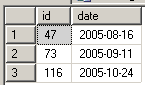SQL Server and T-SQL Development Tutorials Development resources, articles, tutorials, code samples and tools and downloads for ASP.Net, SQL Server, R Script, Windows, Windows Phone, AWS, SAP HANA and ABAP, like SAP UI5, Screen Personas, etc.
 Home Articles News IT Jobs Tools Sample Chapters Trainers Blogs Forums Photos Files

Install SQL Server 2019

# SQL Server Table-Valued Function Example

A SQL Server table-valued function is a user-defined function which returns a table data type as output.
What makes SQL Server table-valued functions more useful in some situations than sql views or sql stored procedures is like every sql function, a t-sql table-valued function also accepts parameters.
Also TVF's (table valued functions) can be referenced in sql SELECT queries just like a SQL Server database table.
These two features plus creating complex t-sql programming logic in user defined functions make a table-valued function powerful than the SQL view and SQL stored procedure objects.

The most well-known use of SQL Table-Valued Function is sql string split function.
Perhaps sql developers can think of the above example as part of this t-sql tutorial.

The following t-sql table-valued function example can be executed on Microsoft SQL Server 2008 R2 sample database AdventureWorks.

``` create function udf_ParseDate (  @date as datetime ) returns @dateinfo table (  id int identity(1,1),  [date] datetime,  [year] int,  [month] smallint,  [day] smallint ) as begin  insert into @dateinfo  select @date, YEAR(@date), MONTH(@date), DAY(@date)  return; end ```

In fact, the above date parser function only returns a single row.
You might prefer to extract Year, Month, and Day date parts within SELECT statements without using a table-valued function.
But please think of udf_ParseDate table-valued function as a starting point in this t-sql tutorial.

``` select  o.SalesOrderID, o.OrderDate,  d.[year], d.[month], d.[day] from Sales.SalesOrderHeader o cross apply dbo.udf_ParseDate(o.OrderDate) d ```

The output of the above sql TVF is as follows.In this t-sql tutorial, I want to give a derived date table example too.
The following t-sql table valued function can be used by t-sql programmers in order to create a table where the start and end date ranges are given as a parameter.

``` create function udf_CreateDateTable (  @mindate as date,  @maxdate as date ) returns @datetable table (  id int identity(1,1),  [date] date ) as begin  declare @i int = 0, @j int = DATEDIFF(dd, @mindate, @maxdate)  while @i <= @j  begin   insert into @datetable   select dateadd(d, @i, @mindate)   set @i = @i + 1  end  return; end ```

T-SQL programmers can use the above user defined table-valued function to find the missing date gaps in SalesOrderHeader table according to the OrderDate column.
Again for this table-valued function example (TVF), I want to use AdventureWorks table.

``` with cte as (  select   [MIN] = MIN(o.OrderDate),   [MAX] = MAX(o.OrderDate)  from Sales.SalesOrderHeader o ) select d.id, d.date from cte cross apply dbo.udf_CreateDateTable(cte.MIN, cte.MAX) d where not exists (  select * from Sales.SalesOrderHeader where OrderDate = d.date ) ```

The following t-sql query which is using TVF - Table Valued Function udf_CreateDateTable will display the dates where no sales order has been created.
Here is the 3 days where no order was created in our AdventureWorks sample database table SalesOrderHeader.Related SQL Resources

SQL Server Articles

SQL Server 2012

SQL Server Tools

SQL Blog

MS SQL Server Forums